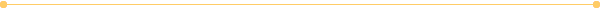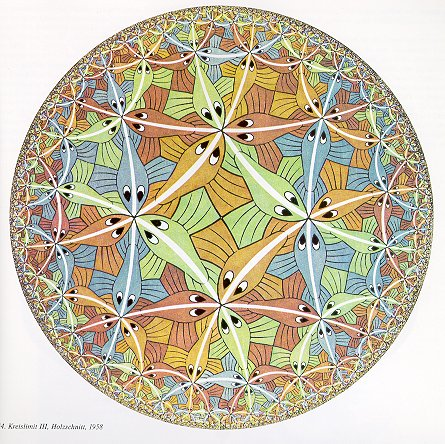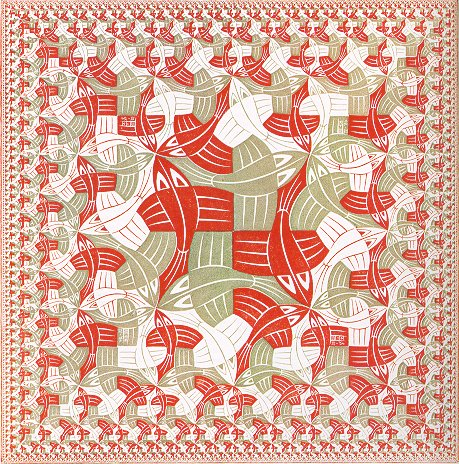Home -> Workshops -> M.C. Escher -> Infinity

# MC Escher: InfinityEscher tried to illustrate the concept of infinity in several works. On the left, he uses what is mathematically known as the "Poincare"disk to tile a two-dimension object with a geometry different from the standard Euclidian one.   Notice how there is again a certain symmetry, involving "curved squares" and "curved triangles", but they are no longer all of the same size.   Toward the edge of the circle, the fish are getting smaller and smaller, and they accumulate near the boundary.   Mathematically, the boundary of the (Poincare) disk has infinite circumference, when measured according to the Poincare distance.This picture shows a similar idea of shapes getting smaller and smaller towards the edge of the square.  It kind of looks like the above picture, but all curves have been replaced by straight lines..Go Back